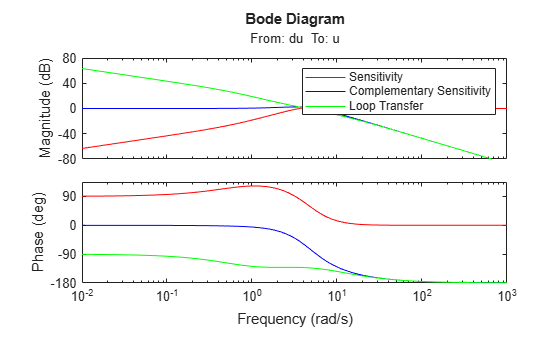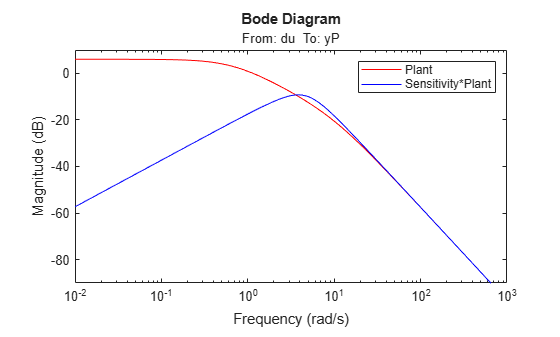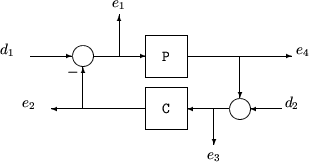loopsens

Sensitivity functions of plant-controller feedback loop

Description

example

loops = loopsens(P,C) computes the multivariable sensitivity, complementary sensitivity, and open-loop transfer functions of the closed-loop system consisting of the controller C in negative feedback with the plant P. To compute the sensitivity functions for the system with positive feedback, use loopsens(P,-C).

Examples

collapse all

Consider PI controller for a dominantly first-order plant, with the closed-loop bandwidth of 2.5 rads/sec. Since the problem is SISO, all gains are the same at input and output.

gamma = 2; tau = 1.5; taufast = 0.1;
P = tf(gamma,[tau 1])*tf(1,[taufast 1]);
tauclp = 0.4;
xiclp = 0.8;
wnclp = 1/(tauclp*xiclp);
KP = (2*xiclp*wnclp*tau - 1)/gamma;
KI = wnclp^2*tau/gamma;
C = tf([KP KI],[1 0]);

Form the closed-loop (and open-loop) systems with loopsens, and plot Bode plots of the sensitivity functions at the plant input.

loops = loopsens(P,C);
bode(loops.Si,'r',loops.Ti,'b',loops.Li,'g')
legend('Sensitivity','Complementary Sensitivity','Loop Transfer')Finally, compare the open-loop plant gain to the closed-loop value of PSi.

bodemag(P,'r',loops.PSi,'b')
legend('Plant','Sensitivity*Plant')Consider an integral controller for a constant-gain, 2-input, 2-output plant. For purposes of illustration, the controller is designed via inversion, with different bandwidths in each rotated channel.

P = ss([2 3;-1 1]);
BW = diag([2 5]);
[U,S,V] = svd(P.d);                % get SVD of Plant Gain
Csvd = V*inv(S)*BW*tf(1,[1 0])*U'; % inversion based on SVD
loops = loopsens(P,Csvd);
bode(loops.So,'g',loops.To,'r',logspace(-1,3,120))
title('Output Sensitivity (green), Output Complementary Sensitivity (red)');Input Arguments

collapse all

Plant, specified as a dynamic system model, control design block, or static gain matrix. P can be SISO or MIMO, as long as P*C has the same number of inputs and outputs.

P can be continuous time or discrete time. If P is a generalized model (such as genss or uss) then loopsens uses the current or nominal value of all control design blocks in P.

Controller, specified as a dynamic system model, control design block, or static gain matrix. The controller can be any of the model types that P can be, as long as P*C has the same number of inputs and outputs. loopsens computes the sensitivity functions assuming a negative-feedback closed-loop system. To compute the sensitivity functions for the system with positive feedback, use loopsens(P,-C).

The loopsens command assumes one-degree-of-freedom control architecture. If you have a two-degree-of-freedom architecture, then construct C to include only the compensator in the feedback path, not any reference channels.

Output Arguments

collapse all

Sensitivity functions of the feedback loop feedback(P,C), returned in a structure having the fields shown in the table below. The sensitivity functions are returned as state-space (ss) models of the same I/O dimensions as C*P. If P or C is a frequency-response-data model, then the sensitivity functions are frd models.

Field

Description

Si

Input-to-plant sensitivity function.

Ti

Input-to-plant complementary sensitivity function.

Li

Input-to-plant loop transfer function.

So

Output-to-plant sensitivity function.

To

Output-to-plant complementary sensitivity function.

Lo

Output-to-plant loop transfer function.

PSi

Plant times input-to-plant sensitivity function.

CSo

Compensator times output-to-plant sensitivity function.

Poles

Poles of the closed loop feedback(P,C). If either P or C is a frequency-response-data model, then this field is NaN.

Stable

1 if nominal closed loop is stable, 0 otherwise. If either P or C is a frequency-response-data model, then this field is NaN.

collapse all

Sensitivity functions

The closed-loop interconnection structure shown below defines the input/output sensitivity, complementary sensitivity, and loop transfer functions. The structure includes multivariable systems in which P and C are MIMO systems.The following table gives the values of the input and output sensitivity functions for this control structure.

Description

Equation

Input sensitivity Si (closed-loop transfer function from d1 to e1)

Si = (I + CP)–1

Input complementary sensitivity Ti (closed-loop transfer function from d1 to e2)

Ti = CP(I + CP)–1

Output sensitivity So (closed-loop transfer function from d2 to e2)

So = (I + PC)–1

Output complementary sensitivity To (closed-loop transfer function from d2 to e4)

To = PC(I + PC)–1

Input loop transfer function Li

Li = CP

Output loop transfer function Lo

Lo = PC